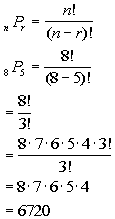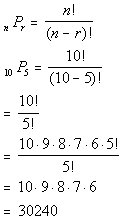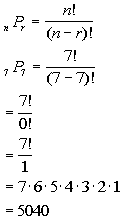TitleCollege Algebra
Answer/Discussion to Practice Problems
Tutorial 56: PermutationAnswer/Discussion to 1a A company issues a questionnaire whereby each employee must rank the 5 items with which he or she is most satisfied.  The items are wages, work environment, vacation time, job security, supervisors, health insurance, break time, and retirement plan. The ranking is to be indicated by the numbers 1, 2, 3, 4 and 5, where 1 indicates the item involving the greatest satisfaction and 5 the least.  In how many ways can an employee answer this questionnaire?

Since we are ranking these items, this means order is important.  So we can use permutations to help us out here.

First we need to find n and r :

 n is the number of items we have to choose from.   What do you think n is in this problem? If you said n is 8 you are correct!!!  There are 8 items listed.   r is the number of items we are ranking at a time.  What do you think r is? If you said r is 5, pat yourself on the back!!  We are ranking the top 5.

Putting this into the permutation formula we get:*n = 8, r = 5       *Expand 8! until it gets to 3! ( which is the ! in den) *Cancel out 3!'s

 If you have a factorial key, you can put it in as 8! divided by 3! and then press enter or =. If you don't have a factorial key, you can simplify it as shown above and then enter it in.  It is probably best to simplify it first, because in some cases the numbers can get rather large, and it would be cumbersome to multiply all those numbers one by one. Wow, this means there are 6720 different ways to rank these items.Answer/Discussion to 1b A key pad lock has 10 different digits, and a sequence of 5 different digits must be selected for the lock to open.  How many key pad combinations are possible?

Since we are counting the number of 5 digit sequences, this means order is important.  So we can use permutations to help us out here.

First we need to find n and r :

 n is the number of digits we have to choose from.   What do you think n is in this problem? If you said n is 10 you are correct!!!  There are 10 digits.   r is the number of digits being used at a time.  What do you think r is? If you said r is 5, pat yourself on the back!!  There are 5 digits in the sequence.

Putting this into the permutation formula we get:*n = 10, r = 5       *Expand 10! until it gets to 5! ( which is the ! in den) *Cancel out 5!'s

 If you have a factorial key, you can put it in as 10! divided by 5! and then press enter or =. If you don't have a factorial key, you can simplify it as shown above and then enter it in.  It is probably best to simplify it first, because in some cases the numbers can get rather large, and it would be cumbersome to multiply all those numbers one by one. Wow, this means there are 30240 different combinations.Answer/Discussion to 1c In how many ways can 7 books be arranged on a shelf?

Since we are arranging these books that means order is important.  So we can use permutations to help us out here.

First we need to find n and r

 n is the number of books we have to choose from.   What do you think n is in this problem? If you said n is 7 you are correct!!!  There are 7 books in this problem.   r is the number of books we are using at a time.  What do you think r is? If you said r is 7, pat yourself on the back!!  We are arranging all 7 books on the shelf.

Putting this into the permutation formula we get:*n = 7, r = 7       *0! = 1    *Expand out 7!

 If you have a factorial key, you can put it in as 7! divided by 0! and then press enter or =. If you don't have a factorial key, you can simplify it as shown above and then enter it in.  It is probably best to simplify it first, because in some cases the numbers can get rather large, and it would be cumbersome to multiply all those numbers one by one. Wow, this means there are 5040 different ways to arrange these books.

Last revised on May 16, 2011 by Kim Seward.
All contents copyright (C) 2002 - 2011, WTAMU and Kim Seward. All rights reserved.